# 69 Results

View
Selected filters:
• Linear Equations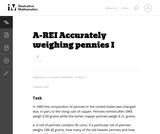Conditions of Use:
No Strings Attached
Rating

This problem involves solving a system of algebraic equations from a context: depending how the problem is interpreted, there may be one equation or two.

Subject:
Mathematics
Algebra
Material Type:
Activity/Lab
Provider:
Illustrative Mathematics
Provider Set:
Illustrative Mathematics
Author:
Illustrative Mathematics
08/15/2012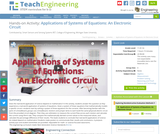Conditions of Use:
No Strings Attached
Rating

Does the real-world application of science depend on mathematics? In this activity, students answer this question as they experience a real-world application of systems of equations. Given a system of linear equations that mathematically models a specific circuit—students start by solving a system of three equations for the currents. After becoming familiar with the parts of a breadboard, groups use a breadboard, resistors and jumper wires to each build the same (physical) electric circuit from the provided circuit diagram. Then they use voltmeters to measure the current flow across each resistor and calculate the current using Ohm’s law. They compare the mathematically derived current values to the measured values, and calculate the percentage difference of their results. This leads students to conclude that real-world applications of science do indeed depend on mathematics! Students make posters to communicate their results and conclusions. A pre/post-activity quiz and student worksheet are provided. Adjustable for math- or science-focused classrooms.

Subject:
Engineering
Algebra
Measurement and Data
Material Type:
Activity/Lab
Provider:
TeachEngineering
Provider Set:
Activities
Author:
Marianne Livezey
11/01/2017Conditions of Use:
No Strings Attached
Rating

Applied Finite Mathematics covers topics including linear equations, matrices, linear programming, the mathematics of finance, sets and counting, probability, Markov chains, and game theory. Endorsed by CollegeOpenTextbooks.org.

Subject:
Finance
Material Type:
Full Course
Textbook
Provider:
Rice University
Provider Set:
Connexions
Author:
Rupinder Sekhon
02/16/2011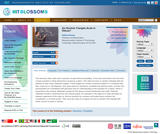Conditions of Use:
Remix and Share
Rating

This learning video deals with a question of geometrical probability. A key idea presented is the fact that a linear equation in three dimensions produces a plane. The video focuses on random triangles that are defined by their three respective angles. These angles are chosen randomly subject to a constraint that they must sum to 180 degrees. An example of the types of in-class activities for between segments of the video is: Ask six students for numbers and make those numbers the coordinates x,y of three points. Then have the class try to figure out how to decide if the triangle with those corners is acute or obtuse.

Subject:
Geometry
Statistics and Probability
Material Type:
Lecture
Provider:
MIT
Provider Set:
MIT Blossoms
Author:
Gilbert Strange
06/02/2012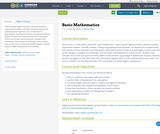Conditions of Use:
No Strings Attached
Rating

Topics include signed numbers, decimal numbers, exponential notation, scientific notation, solving and graphing linear equations, an introduction to polynomials, and systems of linear equations and their graphs. Geometrical topics include lines and angles, closed curves and convex polygons, triangles and similarities, and symmetry and proportion in nature and art. All course content by John McColgan. Content added to OER Commons by Victoria Vidal.

Subject:
Mathematics
Numbers and Operations
Material Type:
Syllabus
Author:
Victoria Vidal
10/10/2019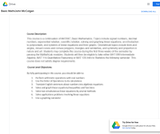Conditions of Use:
No Strings Attached
Rating

This course is a continuation of MAT087, Basic Mathematics. Topics include signed numbers, decimal numbers, exponential notation, scientific notation, solving and graphing linear equations, an introduction to polynomials, and systems of linear equations and their graphs. Geometrical topics include lines and angles, closed curves and convex polygons, triangles and similarities, and symmetry and proportion in nature and art. Students may complete this course during the first three weeks of the semester by passing the MyMathLab modules. Students will then be eligible to take either MAT 099 Intermediate Algebra, MAT 114-Quantitative Reasoning or MAT 120-Intro to Statistics the following semester. This course does not satisfy degree requirements.

Subject:
Mathematics
Material Type:
Full Course
Provider:
Roxbury Community College
Author:
John McColgan
05/15/2019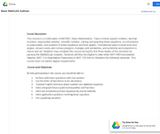Conditions of Use:
No Strings Attached
Rating

This course is a continuation of MAT087, Basic Mathematics. Topics include signed numbers, decimal numbers, exponential notation, scientific notation, solving and graphing linear equations, an introduction to polynomials, and systems of linear equations and their graphs. Geometrical topics include lines and angles, closed curves and convex polygons, triangles and similarities, and symmetry and proportion in nature and art. Students may complete this course during the first three weeks of the semester by passing the MyMathLab modules. Students will then be eligible to take either MAT 099 Intermediate Algebra, MAT 114-Quantitative Reasoning or MAT 120-Intro to Statistics the following semester. This course does not satisfy degree requirements.

Subject:
Mathematics
Material Type:
Full Course
Provider:
Northern Essex Community College
Author:
Jim Sullivan
05/15/2019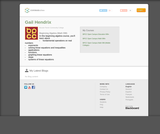Rating

In this beginning algebra course, you'll learn about
fundamental operations on real numbers
exponents
solving linear equations and inequalities
applications
functions
graphing linear equations
slope
systems of linear equations

Subject:
Algebra
Material Type:
Full Course
Lecture
Lesson
Module
Provider:
Bossier Parish Community College
Author:
Gail Hendrix
10/16/2017Rating

This lesson unit is intended to help teachers assess how well students are able to create and solve linear equations. In particular, the lesson will help you identify and help students who have the following difficulties: solving equations with one variable and solving linear equations in more than one way.

Subject:
Algebra
Material Type:
Assessment
Lesson Plan
Provider:
Shell Center for Mathematical Education
U.C. Berkeley
Provider Set:
Mathematics Assessment Project (MAP)
04/26/2013Conditions of Use:
Remix and Share
Rating

This task was developed by high school and postsecondary mathematics and health sciences educators, and validated by content experts in the Common Core State Standards in mathematics and the National Career Clusters Knowledge & Skills Statements. It was developed with the purpose of demonstrating how the Common Core and CTE Knowledge & Skills Statements can be integrated into classroom learning - and to provide classroom teachers with a truly authentic task for either mathematics or CTE courses.

Subject:
Health, Medicine and Nursing
Algebra
Ratios and Proportions
Material Type:
Activity/Lab
Lesson Plan
Provider:
National Association of State Directors of Career Technical Education Consortium
Provider Set:
Career Technical Education
07/27/2012Conditions of Use:
Remix and Share
Rating

This task was developed by high school and postsecondary mathematics and health sciences educators, and validated by content experts in the Common Core State Standards in mathematics and the National Career Clusters Knowledge & Skills Statements. It was developed with the purpose of demonstrating how the Common Core and CTE Knowledge & Skills Statements can be integrated into classroom learning - and to provide classroom teachers with a truly authentic task for either mathematics or CTE courses.

Subject:
Health, Medicine and Nursing
Algebra
Material Type:
Activity/Lab
Lesson Plan
Provider:
National Association of State Directors of Career Technical Education Consortium
Provider Set:
Career Technical Education
07/27/2012Conditions of Use:
Remix and Share
Rating

This task was developed by high school and postsecondary mathematics and health sciences educators, and validated by content experts in the Common Core State Standards in mathematics and the National Career Clusters Knowledge & Skills Statements. It was developed with the purpose of demonstrating how the Common Core and CTE Knowledge & Skills Statements can be integrated into classroom learning - and to provide classroom teachers with a truly authentic task for either mathematics or CTE courses.

Subject:
Health, Medicine and Nursing
Algebra
Functions
Material Type:
Activity/Lab
Lesson Plan
Provider:
National Association of State Directors of Career Technical Education Consortium
Provider Set:
Career Technical Education
07/26/2012Conditions of Use:
No Strings Attached
Rating

This task presents a real-world problem requiring the students to write linear equations to model different cell phone plans. Looking at the graphs of the lines in the context of the cell phone plans allows the students to connect the meaning of the intersection points of two lines with the simultaneous solution of two linear equations.

Subject:
Mathematics
Material Type:
Activity/Lab
Provider:
Illustrative Mathematics
Provider Set:
Illustrative Mathematics
Author:
Illustrative Mathematics
05/01/2012Rating

This lesson unit is intended to help teachers assess how well students are able to classify solutions to a pair of linear equations by considering their graphical representations. In particular, this unit aims to help teachers identify and assist students who have difficulties in: using substitution to complete a table of values for a linear equation; identifying a linear equation from a given table of values; and graphing and solving linear equations.

Subject:
Algebra
Material Type:
Assessment
Lesson Plan
Provider:
Shell Center for Mathematical Education
U.C. Berkeley
Provider Set:
Mathematics Assessment Project (MAP)
04/26/2013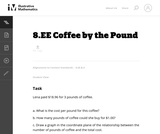Conditions of Use:
No Strings Attached
Rating

Students are exptected to identify the slope of the line with the unit rate in this real world problem.

Subject:
Mathematics
Material Type:
Activity/Lab
Provider:
Illustrative Mathematics
Provider Set:
Illustrative Mathematics
Author:
Illustrative Mathematics
05/01/2012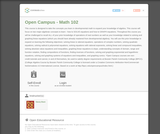Rating

This course is designed to take the concepts you learn in developmental math to expand your knowledge of algebra. This course will focus on two major algebraic concepts to learn - how to SOLVE equations and how to GRAPH equations. Throughout this course you will be challenged to recall ALL of your prior knowledge of operations of real numbers as well as your knowledge related to solving and graphing linear equations (which you should have already mastered from developmental algebra). You will use this prior knowledge to expand on learning the following objectives: solving linear & rational equations. operations of complex numbers, solving quadratic equations, solving radical & polynomial equations, solving equations with rational exponents, solving linear and compound inequalities, solving absolute value equations and inequalities, graphing linear equations & slope, understanding concepts of domain, range and function notation, finding compositions of functions, finding inverses of functions, solving and graphing exponential and logarithmic equations, solving and graphing systems of equations and inequalities, and graphing conics.

*Open Campus courses are non-credit tutorials and cannot, in and of themselves, be used to satisfy degree requirements at Bossier Parish Community College (BPCC). (College Algebra Course by Bossier Parish Community College is licensed under a Creative Commons Attribution-NonCommercial-NoDerivatives 4.0 International License. Based on a work at http://bpcc.edu/opencampus/index.html.)

Subject:
Mathematics
Algebra
Material Type:
Full Course
Provider:
Bossier Parish Community College
Author:
Stacey Black
10/11/2017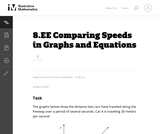Conditions of Use:
No Strings Attached
Rating

This task provides the opportunity for students to reason about graphs, slopes, and rates without having a scale on the axes or an equation to represent the graphs. Students who prefer to work with specific numbers can write in scales on the axes to help them get started.

Subject:
Mathematics
Material Type:
Activity/Lab
Provider:
Illustrative Mathematics
Provider Set:
Illustrative Mathematics
Author:
Illustrative Mathematics
05/01/2012Conditions of Use:
No Strings Attached
Rating

This task involves solving equations with rational coefficients, and requires students to use the distributive law ("combine like terms"). The equation also provides opportunities for students to observe structure in the equation to find a quicker solution, as in the second solution presented.

Subject:
Mathematics
Material Type:
Activity/Lab
Provider:
Illustrative Mathematics
Provider Set:
Illustrative Mathematics
Author:
Illustrative Mathematics
05/01/2012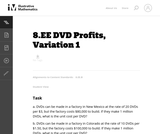Conditions of Use:
No Strings Attached
Rating

This task requires students to work with unit rates.

Subject:
Mathematics
Material Type:
Activity/Lab
Provider:
Illustrative Mathematics
Provider Set:
Illustrative Mathematics
Author:
Illustrative Mathematics
05/01/2012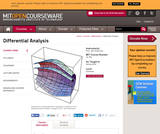Conditions of Use:
Remix and Share
Rating

Fall: Fundamental solutions for elliptic, hyperbolic and parabolic differential operators. Method of characteristics. Review of Lebesgue integration. Distributions. Fourier transform. Homogeneous distributions. Asymptotic methods. Spring: Sobolev spaces. Fredholm alternative. Variable coefficient elliptic, parabolic and hyperbolic linear partial differential equations. Variational methods. Viscosity solutions of fully nonlinear partial differential equations. The main goal of this course is to give the students a solid foundation in the theory of elliptic and parabolic linear partial differential equations. It is the second semester of a two-semester, graduate-level sequence on Differential Analysis.

Subject:
Engineering
Material Type:
Full Course
Provider:
MIT
Provider Set:
MIT OpenCourseWare
Author:
Viaclovsky, Jeffrey Alan